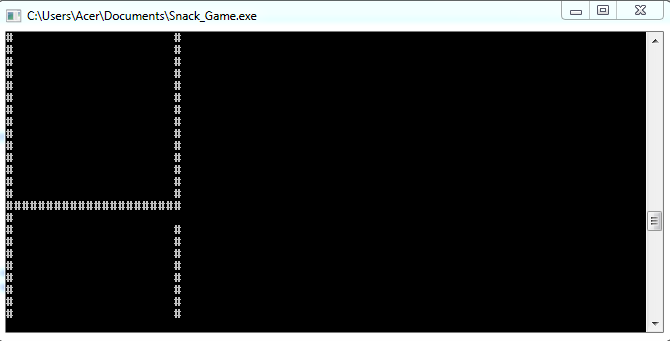# No error while compiling, but the result does not work well [C++]

I am sorry, i don’t understand what is wrong with my code. I follow along the tutorial on YouTube, when i compile the code there is no error, but when i run it, it does not work very well.

Here is the result which not work well:I want to put the picture of how the result should be, but cause i am new here so i can’t put two picture. Maybe you all can visit in this tutorial which at the end of the video is the result should be, please.

Here is my code:

``````#include <iostream>
#include <stdlib.h>

using namespace std;
bool gameOver;
const int width = 20;
const int height = 20;
int x, y, fruitX, fruitY, score;
enum eDirecton { STOP = 0, LEFT, RIGHT, UP, DOWN};
eDirecton dir;

void Setup(){
gameOver = false;
dir = STOP;
x = width / 2;
y = height / 2;
fruitX = rand() % width;
fruitY = rand() % height;
score = 0;
}
void Draw(){
system("cls");
for (int i = 0; i < width+2; i++){
cout <<"#";
cout << endl;

for (int i = 0; i < height; i++){

for (int j = 0; j < width; j++)
{
if (j == 0)
cout << "#";

cout << " ";

if (j == width - 1)
cout << "#";
}
cout << endl;
}
for (int i = 0; i < width+2; i++)
cout << "#";
cout << endl;
}
}
void Input(){

}
void Logic()
{

}
int main()
{
Setup();
while (!gameOver)
{
Draw();
Input();
Logic();
//Sleep(10)
}
return 0;
}

Thanks for helping me.``````

It seems to work ok. I found I had to scroll up when viewing it on the test site at https://www.onlinegdb.com/online_c++_compiler

sorry, my bad. After viewing the expected output, I realised your problem is on line 31. There is no open bracket in the tutorial, so the loop should only affect the following line. You need to remove this bracket and the end closing bracket.